Scroll

# Correction (Weighting) Factors Applied in Tables I, II & III

Follow
 Summary This article is one in a series of articles that will go through examples of using table arithmetic syntax new to Askia 5.3.5.0. This article demonstrates the post processing or 'Cleaning script' to correct certain brand's figures, for certain waves, by multiplying them by predetermined factors. Applies to Askia Analyse. Written for Researchers, Developers, Data Processors. Keywords Post processing, Cleaning script, Conditional formatting, Table arithmetic, Forloops, Coordinates, data, Calculation arithmetic, Weighting, Calibration, Correction, Factor, Coefficient.

Example I

The .qes file and portfolio containing the example discussed is attached: Table Arithmetic Examples 6.rar

We have a table set-up (WeightCheck - Original) as follows: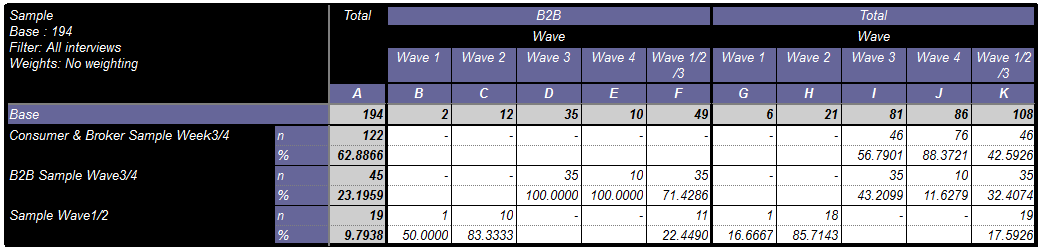The requirement is to multiply all % for B2B Sample Wave3/4 by 0.5 and then create a total % and base for all types (Consumer, Broker & B2B) including this adjustment. This can be seen in the tab definition (WeightCheck - with COEFF)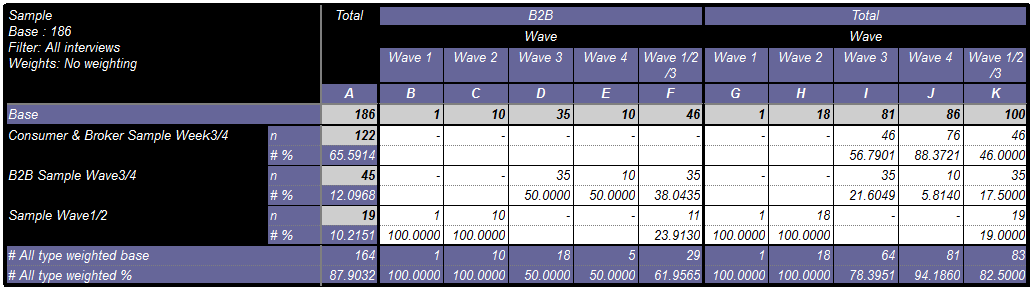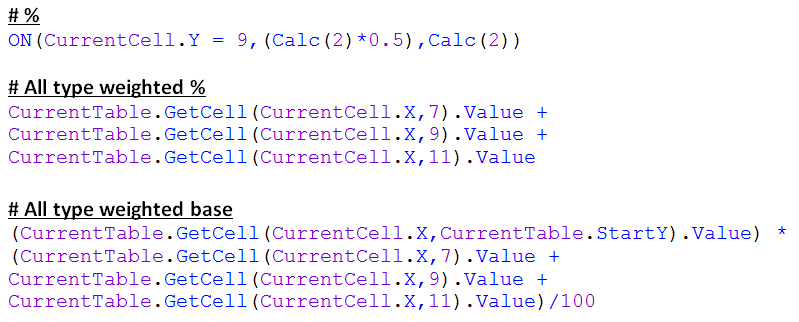You may be wondering what is Calc(2) in the first portion of syntax above.

It's actually one of the calculations that are already present in our list of calculations. It doesn't appear in our table because it has the option 'Hide this calculation but leave it available for arithmetic or conditional formatting'. We're simply saying at this point: "When we are on the 9th row of our table, return the % multiplied by 0.5, otherwise, return the % calculation."We then want a final table which will display only the base row, and adjusted (weighted) % and base rows. This is seen in the tab definition (WeightCheck - with COEFF (Final)).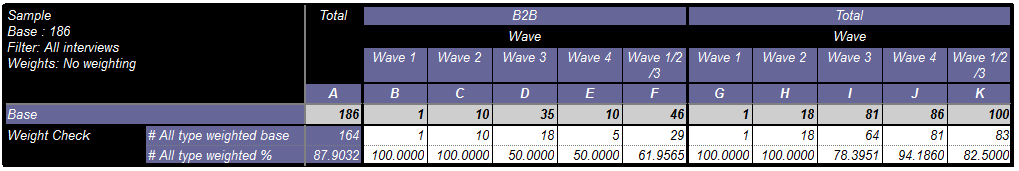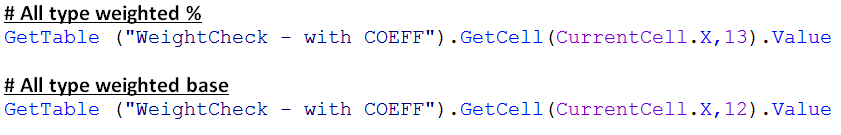There will be more a complex variation of this example which will deal with recognising the category caption so that we don't need to use hard coded values in our Y co-ordinates. This will be more advanced syntax but will allow for more flexibility with the resultant table.

Example II

The .qes file and portfolio containing the example discussed is attached: Table Arithmetic Examples 8.rar.

Here the requirement is to multiply **B2B** figures by a factor of 2 (for wave 3 onwards) and make sure that when this has been done, these are incorporated into the column total Counts and %. We start with the first table below.And were looking to create the table directly above with our cleaning script. The colours are set in the script as well just to demonstrate which areas of the table we are looking at. They can be switched off as demonstrated in the example file.

Here's the syntax with helpful comments to step through how we achieve this:Adding rows, edges or columns will not affect what we are required to achieve here. If we only wanted to weight waves 3 and 4 and then introduce additional waves in the rows then we would need to make an adjustment to our y co-ordinate ranges defined in the syntax.

Users will find this useful for bespoke weighting & correction of figures or bespoke calculations in their tables.

Example III

The .qes file and portfolio containing the example discussed is attached: Table Arithmetic Examples 4.rar.

We have a table of insurance brands in our rows, Wave in columns and sample type in edges.Tab definition: (No cleaning)

The requirement here is to multiply certain figures by corrective factors

• Rows which contain the text ‘AXA’, its figures for Wave 3 & 4 Broker should be multiplied by 0.5
• Rows which contain the text ‘Churchill’, its figures for Wave 3 & 4 Broker should be multiplied by 0.75
• Rows which contain the text ‘Zurich’, its figures for Wave 1 & 2 Broker should be multiplied by 1.5

The result is found in the tab definition: (Caption based cleaning & calculations)In the tab template for this definition (Clean_SA) you will find the cleaning script applied (Settings > Sorting > Cleaning script).

Remember here that CurrentTable.StartY = 5  and that CurrentTable.MaxY = 9This example demonstrates how to manipulate figures based on text in row stubs. There will be a, more advanced, follow-up example showing how to manipulate data based on a combination of row and column stub text.